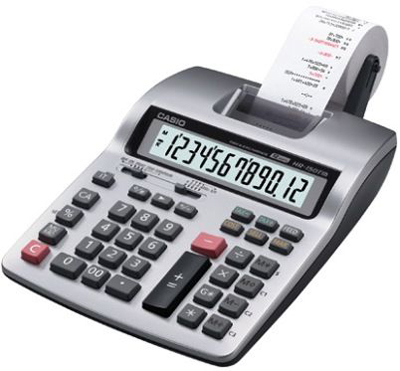We all know what calculators are and have been using them to quickly and accurately find the solution to certain mathematical tasks. These time-saving tools comes in many different types, such as graphics calculators, scientific calculators, programmable calculators, etc. all designed for a particular application. These universal tools are an inevitable tool of many daily operations in the business world and to choose the right calculator for your job, you must know what differs one model from the other.

# Basic, Hand-Held CalculatorThe basic, hand-held calculator is the most common type and can be found in every home. It can fit a pocket, uses solar energy and is perfect for calculating basic math tasks. Usually, this calculator comes with only a few functions and a one-line LED display. Since it is usually only used for adding, subtracting, dividing and multiplying, it is known as a four-function calculator. There are some basic calculator models with a large, easily readable display and a back-up battery. Some can even do more functions, such as calculating taxes, discount amounts, square roots, etc.

# Scientific CalculatorScientific calculators provide a plenty of highly-advanced calculations, statistics, analysis, scientific data plotting, conversions and much more. So far, scientific calculators are the most advanced calculator models and they are especially efficient for general math, engineering and algebra. College students, teachers, engineers and scientists all use scientific calculators for performing all sorts of complicated math functions.

# Graphics CalculatorGraphics calculators are the most common form of scientific calculators. These calculators provide over a hundred of different math functions and are used in many different applications. The graphics calculators are able to perform any calculation, including solving, sketching and graphing complex statistics. They are considered mini-computers and are must-have in math, science and technical fields. The latest graphics calculators feature high-resolution LCD screens that are easy to read and incredibly fast CPUs, able to perform the most complex graphics and calculations. Also, they can be connected with a computer for even greater functionality, as well as a flash memory for storage of data and applications.

# Printing CalculatorBefore computers were present in every home, printing calculators were widely used. Beside displaying the result on a screen, these calculators print the results in ink onto a paper. They also come with one more roll of paper inside the printer, which is used to keep a written record of all calculations that have been made. Most printing calculators are able to print in two colours, black and red, in order to easily identify positive and negative values. Printing calculators can be classified into three groups: compact, desktop and thermal models.